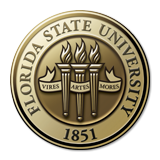Florida State University
FSUMATH# Department of Mathematics

### Mathematics WWW Virtual Library

#### Virtual Library Home : Online BooksRecently Added ( 0 )## Calculus, AnalysisMultivariable Calculus by George Cain & James Herod (used at Georgia Tech)Introduction to Tensor Calculus and Continuum Mechanics by John H. HeinbockelComplex Analysis by George CainAn Introduction to Fourier Theory by Forrest HoffmanAn Introduction to C*-Algebras by Pierre de la Harpe, Vaughan Jones. (The page is in French but the book is in English; advanced)## AlgebraAbstract Algebra: The Basic Graduate Year by Robert B. AshElements of Abstract and Linear Algebra by Edwin H. Connell (used at U of Miami, Coral Gables)Elementary Linear Algebra by Keith MatthewsLinear Algebra by Jim HefferonSÃ©minaire de GÃ©ometrie AlgÃ©brique Entire collection (vols. 1-7) scanned into jpeg files.## GeometryAn Introduction to Riemannian Geometry (postscript file)Euclid's ElementsNatural operations in differential geometry by Ivan Kolar, Jan Slovak and Peter W. Michor (Springer Verlag) (this is from the EMIS collection (see below); advanced)## OtherBasic Concepts of Mathematics An online book that helps the student make the transition from purely manipulative to rigorous mathematics.Hilbert Space Methods for Partial Differential Equations by R. E. ShowalterIntroduction to Probability by Dimitri P. Bertsekas and John N. TsitsiklisIntroduction to Probability by Grinstead, Snell (AMS)Linear Methods of Applied Mathematics by Evans M. Harrell II and James V. HerodNumerical Recipes (Cambridge Univ. Press)A Problem Course in Mathematical Logic by Stefan BilaniukTemplates for the solution of linear systems: building blocks for iterative methods (SIAM)Chaitin: The Limits of Mathematics## Book Collections by an authorAllen Hatcher (Cornell)Herb Wilf (U Penn)George Cain (Georgia Tech)Igor Dolgachev (U Mich)Algebra: Abstract and Concrete Introduction to abstract algebra at the beginning graduate or upper level undergraduate level.An Introduction to the Theory of Numbers This book, which presupposes familiarity only with the most elementary concepts of arithmetic (divisibility properties, greatest common divisor, etc.), is an expanded version of a series of lectures for graduate student on elementary number theory. Topics include: Compositions and Partitions; Arithmetic Functions; Distribution of Primes; Irrational Numbers; Congruences; Diophantine Equations; Combinatorial Number Theory; and Geometry of Numbers.Introduction to ProbabilityMathematical Analysis I This text covers the basic topics of undergraduate real analysis including metric spaces, open and closed sets, convergent sequences, function limits and continuity, compact sets, sequences and series of functions, power series, differentiation and integration, Taylor's theorem, total variation, rectifiable arcs, and sufficient conditions of integrability.Open Problems in Topology A collection of 1100 open problems in topology edited by Jan van Mill and George M. Reed. Cumulative status reports on these problems appear periodically under the same title in Topology and its Applications.